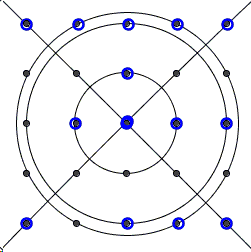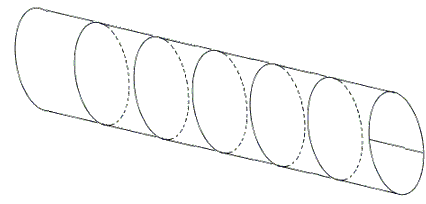# Puzzle of 25 Grid Points and 5 Circles

### Problem

Given a 5×5 square grid.Find five circles so that they pass through each of the 25 grid points at least once.

### A Story

In his book Mathematical Delights Ross Honsberger tells about a letter Professor Liong-shin Hahn received from a mathematics teacher. The latter posed to his student the problem as it was stated above.

One of the students reported the following solution:explaining that a straight line is nothing but a circle with an infinite radius. When the teacher insisted that the task was to find circles with finite radii, the student surprised the teacher with another diagram:To teacher's objections the student rolled the diagram into a cylinder, making all circles of finite radius:Naturally, that was not what the teacher had in mind. But what was it?

The applet below illustrates the problem. It may be helpful in finding the solution.

### Solution

Here's one possible solution:And here's another one:Here's a solution found by David Radcliffe:Are there other, essentially different solutions?

### Acknowledgement

An older Java based variant of this page appears on a separate page.

Alexandre Muñiz (on twitter) suggested there is a way of covering a 5×6 grid with 5 circles. I placed the puzzle on a separate page.

### References

1. R. Honsberger, Mathematical Delights, MAA, 2004, 155-158